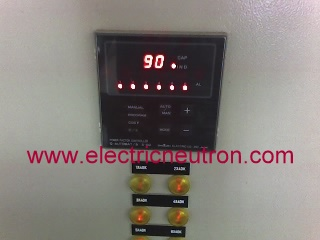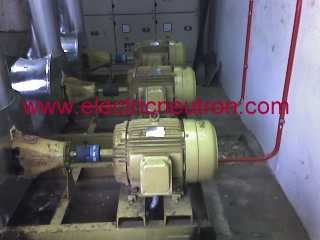# Power Factor

For the simple and easy understanding,power factor is the Alternating current ( AC ) value of electrical efficiency.The ratio of true power flow into load of apparent power.Power Factor is the ratio of Working Power to Apparent Power.If equipment or machine running in maximum efficiency,we can save the electric cost and keep the long life span.

The range of power factor is between 0 and 1.Normally it state in percentage value e.g :  85 % pf.In power distribution,It in two condition of power factor,Leading or Lagging otherwise Inductive or Capacitive.They required the minimum level of power factor ( pf ) between 0.80 until 0.85 ( 80% – 85% ).If not they imposed surcharge to the owner of installation.

## What is power factor?## Why need to set the minimum level for power factor?

Power generator actually used the mechanical force to generate the electricity.So,they need expense the cost for it e.g diesel,gases,coal,water etc.

If user not maintained the minimum level,distributor need add more cost for generated the electricity power for achieve the  power demand.In other word,they have to expense more cost for the unnecessary  and efficient power supply demand.

Actually,we can categories three (3 ) types of power :-

### 1) True Power

– It measured in kilowatt ( kW ).This is the true power for electrical device to operated.

### 2) Reactive Power

– It also known as Magnetizing power.It measured in kilovars ( kVAR ).The inductive device need this power to generate the necessary of flux to operation needed.

### 3) Apparent power

– It is the two element in the circuit,current ( I ) and the Voltage ( V ).This power is combination from Working power ( kW ) with Reactive power ( kVAR ).It measured in kilo volt ampere ( kVA )

So…from this three ( 3 ) types of power,we can conclude the formula for power factor ( PF ) as below :

# PF = kW / kVA

## What is factor for low power factor?

The main factor for low power factor is the inductive load,in other word it is the reactive power ( kVAR ).This load generate the electromagnetic field.The example of inductive load equipment or device :

• Transformer
• Motor
• High Intensive Discharge Lighting ( HID )
• Rectifier
• Solenoid
• Relays
• ElectromagnetThis site uses Akismet to reduce spam. Learn how your comment data is processed.